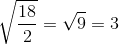# SAT II Chemistry : Effusion

## Example Questions

### Example Question #1 : Solutions And States Of Matter

How much faster is the rate of effusion of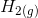than the rate of effusion of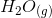?By Graham's Law,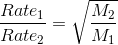. The molar mass of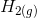is 2 g/mol and the molar mass ofis 18 g/mol. Thus,